GRE Subject Test: Chemistry : Terpene Chemistry

Example Questions

Example Question #1 : Lipids

Which of the following molecule is a type of terpene?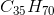because it is derived from a pentene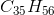because it is derived from a pentenebecause it is derived from an isoprenebecause it is derived from an isoprenebecause it is derived from an isoprene

Explanation:

Terpenes are special classes of lipids that are derived from isoprene units. An isoprene unit’s molecular formula is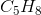; therefore, terpenes must contain a molecular formula that is derived from aempirical formula.

The molecular formulaconsists of seven isoprene units (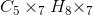=); therefore,is a type of terpene.

Pentene is an alkene with the molecular formula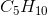.(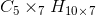) is derived from pentene units; however, terpenes do not have pentene units.

All GRE Subject Test: Chemistry Resources